# Author:

Chao Li Shunsuke Managi

# 1.Overview

This is a basic introduction. If you have been familiar with geographically weighted regressions (GWR), you can skip this part and directly go to the sections about the Functions. If not? Well, you are going to get a funny story.

## 1.1 What is Geographically Weighted Panel Regression (GWPR)

Generally, this algorithm mainly solves the problem of residuals from panel regressions clustering spatially. Basically, the GWPR is a type of weighted regression, but the only difference between Weighted Least Square (WLS) and GWPR is where the weights come from. In following sections, we will provide more information about the weights.

## 1.2 Why we need to use GWPR

As we have mentioned that, sometimes, the residuals cluster spatially, but the widely used method, Ordinary least squares (OLS), assume that the residuals distribute randomly, even on the maps. We give an cross-sectional example using the data in the package “GWPR.light”, since the cross-sectional example is easy to read.

library(GWPR.light)
library(sp)
library(tmap)
#> Warning: 程辑包'tmap'是用R版本4.1.3 来建造的
data("California")
data("TransAirPolCalif")
formula.GWPR <- pm25 ~ co2_mean + Developed_Open_Space_perc + Developed_Low_Intensity_perc +
Developed_Medium_Intensity_perc + Developed_High_Intensity_perc +
Open_Water_perc + Woody_Wetlands_perc + Emergent_Herbaceous_Wetlands_perc +
Deciduous_Forest_perc + Evergreen_Forest_perc + Mixed_Forest_perc +
Shrub_perc + Grassland_perc + Pasture_perc + Cultivated_Crops_perc +
pop_density + summer_tmmx + winter_tmmx + summer_rmax + winter_rmax
TransAirPolCalif <- dplyr::filter(TransAirPolCalif, year == 2001)
lm.2001 <- lm(formula = formula.GWPR, TransAirPolCalif)
summary(lm.2001)
#>
#> Call:
#> lm(formula = formula.GWPR, data = TransAirPolCalif)
#>
#> Residuals:
#>     Min      1Q  Median      3Q     Max
#> -4.8287 -0.7795 -0.0499  0.7059  3.9260
#>
#> Coefficients:
#>                                     Estimate Std. Error t value Pr(>|t|)
#> (Intercept)                       -1.263e+02  6.333e+01  -1.995 0.053454 .
#> co2_mean                           3.453e+00  1.441e+00   2.396 0.021733 *
#> Developed_Open_Space_perc          2.308e-01  3.062e-01   0.754 0.455674
#> Developed_Low_Intensity_perc       1.163e-01  7.643e-01   0.152 0.879920
#> Developed_Medium_Intensity_perc    4.762e-01  7.783e-01   0.612 0.544383
#> Developed_High_Intensity_perc     -2.806e+00  2.197e+00  -1.278 0.209329
#> Open_Water_perc                   -1.361e-02  1.242e-01  -0.110 0.913294
#> Woody_Wetlands_perc               -5.658e+00  1.520e+00  -3.723 0.000653 ***
#> Emergent_Herbaceous_Wetlands_perc  5.911e-04  1.915e-01   0.003 0.997553
#> Deciduous_Forest_perc             -2.392e-01  3.316e-01  -0.721 0.475234
#> Evergreen_Forest_perc              1.220e-01  8.920e-02   1.367 0.179836
#> Mixed_Forest_perc                 -2.076e-01  1.279e-01  -1.623 0.113014
#> Shrub_perc                         1.637e-01  8.684e-02   1.885 0.067341 .
#> Grassland_perc                     1.182e-01  7.712e-02   1.532 0.133914
#> Pasture_perc                       1.097e+00  5.122e-01   2.142 0.038867 *
#> Cultivated_Crops_perc              1.782e-01  8.407e-02   2.120 0.040789 *
#> pop_density                       -5.025e+00  3.157e+00  -1.592 0.119975
#> summer_tmmx                       -5.369e-01  2.775e-01  -1.935 0.060675 .
#> winter_tmmx                        1.039e+00  2.891e-01   3.592 0.000948 ***
#> summer_rmax                       -1.761e-01  6.803e-02  -2.589 0.013694 *
#> winter_rmax                        5.998e-02  9.673e-02   0.620 0.538986
#> ---
#> Signif. codes:  0 '***' 0.001 '**' 0.01 '*' 0.05 '.' 0.1 ' ' 1
#>
#> Residual standard error: 1.769 on 37 degrees of freedom
#> Multiple R-squared:  0.8337, Adjusted R-squared:  0.7439
#> F-statistic: 9.277 on 20 and 37 DF,  p-value: 4.693e-09
resid.lm <- cbind(lm.2001$residuals, TransAirPolCalif$GEOID)
colnames(resid.lm) <- c("resid", "GEOID")
#>        resid GEOID
#> 1 -1.3829415  6091
#> 2 -2.5769982  6067
#> 3 -0.2371560  6083
#> 4  1.1396837  6009
#> 5 -0.7800447  6111
#> 6  0.6832170  6037
to_show <- sp::merge(California, resid.lm, by = "GEOID")
tm_shape(to_show) + tm_polygons(col = 'resid')
#> Warning in sp::proj4string(obj): CRS object has comment, which is lost in output
#> Variable(s) "resid" contains positive and negative values, so midpoint is set to 0. Set midpoint = NA to show the full spectrum of the color palette.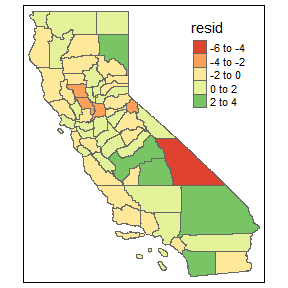Note: This is just an example about the residuals spatially clustering. Maybe, not so clear to convince you, so we need use Moran’s I test to statistically confirm this situation. We will discuss that part in the Function section.

## 1.3 How we can perform GWPR

Before the package “GWPR.light” had been created, it was a little bit complicated. You need to know how to transform the raw data. Of course, among the well-known models, between panel regression, pooling regression, first difference and fixed effects model are not difficult to apply the data transformation. Then, the algorithm divides the whole sample into numerous subsamples according to a specific rules (we will discuss in the following section). The individual regressions of the subsamples are performed to estimate the local coefficients. However, the random effects model makes us have a headache. Theta $$\theta$$ is really hard to confirm. We do not know whether they change in each subsample. If we remove the individual effects based on the global $$\theta$$, then it may reduce accuracy, though it works. If we try to remove the individual effects in each subsample manually, it is impossible unless you are willing to pay several months or more. So:

library(GWPR.light)

# 2 Functions

## 2.1 bw.GWPR(): who is your neighborhoods?

Who is the neighborhoods of the specific point is really hard to define. In the Beenstock’s and Fotheringham’s books(ref. 1 & 2), if the distance ($$d$$) between point A and point B is within a certain distance ($$h$$), they could be deemed as the neighborhood of each other, because they have a spatial relationship ($$a$$). The Equations are as follows: $a_{ik}= \begin{cases} 1& d_{ik} < h\\ 0& \text{otherwise} \end{cases}$ where $$d_{ik}$$ is the distance between the locations of observations $$i$$ and $$k$$. It is also possible to relate $$d_{ik}$$ to $$a_{ik}$$ with a continuous function. Currently, this equation is widely used: $a_{ik} = \begin{cases} [1 - (d_{ik}/h)^2]^2 & d_{ik} < h\\ 0& \text{otherwise} \end{cases}$ In fact, numerous research that the closer the objects are, the more similar they are. Since the relationship have been normalized from 0 to 1. Therefore, here, we can say the weights ($$W$$) of those relationships are the same: $W_{ik} = a_{ik}$

### 2.1.1 Choosing $$h$$: the kernel bandwidth

bw.GWPR(formula = formula, data = data, index = index, SDF = SDF,
adaptive = F, p = 2,bigdata = F, upperratio = 0.25,
effect = "individual", model = c("pooling", "within", "random"),
random.method = "swar", approach = c("CV","AIC"), kernel = "bisquare",
longlat = F, doParallel = F, human.set.range = F,
h.upper = NULL, h.lower = NULL)

There is a setting named “approach”, including two selections: one is “CV” and the other is “AIC”. They are two criteria to calibrate the bandwidth. In “CV” method, when the sum of squared errors arrives at the minimum, the bandwidth is optimal. Basically, the sum of squared errors is written as: $SS(h) = \sum_i\{y_i - \hat{y_i}(h)\}^2$ However, when $$h$$ varies, $$i$$ also changes. Possibly, this function is monotonically increasing.

h = 1:10 # just an example, not truth.
SS = h^2
plot(h, SS, col = "red")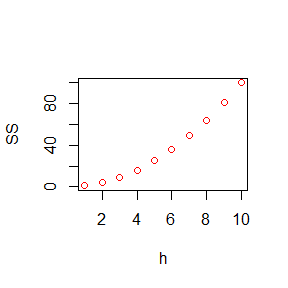To solve this issue, the $$SS$$ function changes as follows (ref. 2): $CVSS(h) = \frac{n \sum_i\{y_i - \hat{y_i}(h)\}^2} {(n-p+1)^2}$ where $$n$$ is the numbers of observations, $$p$$ is the number of the estimated parameters. Furthermore, the “AIC” criterion also has the same issue. It has changed in our calculation: $AIC(h) = 2nln(sd) + 2ln(2\pi) + \frac{n\times [tr(hat) + n]}{[n - 2 - tr(hat)]}$ where $$sd$$ is the standard deviation of residuals, and $$hat$$ is the hat matrix. Now, you get the fixed distance bandwidth!:)

### 2.1.2 Adaptive and Fixed Distance Bandwidth

Sometimes, some researches assume the numbers of neighborhoods is fixed. In other words, a specific point always has the $$n$$ neighborhoods, no matter how far they are. Here, we are going to use the adaptive distances bandwidth. The $$h$$ is not a fixed number, but depends on the furthest neighborhood. If you have this request, you need to select the “adaptive” as TRUE.

### 2.1.3 “bigdata” Setting

Our calibration process normally start from the golden ratio of range. In this way, if your dataset is huge, in the beginning, the calculation is really time-consuming. However, according to our experience, the bandwidth is seldom large. Therefore, you could set “bigdata” as TRUE and reset upper boundary.

### 2.1.4 “human.set.range” Setting

If you have run this function for several times and you know the potential optimal range of bandwidth, you can set “human.set.range” as TRUE to cut down the calculation time. Then, you must also set h.upper and h.lower both. Otherwise, errors.

Note: This setting is super important.
In some remote sensing research, we find the relationship between bandwidth and CV or AIC score is not U-shape. So, we recommend to use gradient increment selection to calibrate the optimal bandwidth. The user need to set the upper boundary of the gradient increment selection (GI.upper), the lower boundary (GI.lower), and the step length of the increment (GI.step). If it is an analysis on grid data, we recommend the GI.step is the spatial resolution.

### 2.1.6 “model” Setting

Now, this version of “GWPR.light” can figure our fixed effects model (“within”), pooling regression (“pooling”), and random effects models (“random”), internally. However, if you perform the basic transformation, we can also perform first difference model and between model. For first difference model, you need to confirm your data is balanced panel. Then, please calculate the difference between two continuous period of each variable in every individual. Reset the “time” index, put them into the function and set the model = “pooling”. Here we go! For between model, you need to directly calculation the mean of each variables in every individual. Then, reset the “time” index, put them into the function and set the model = “pooling”. You got it. Unfortunately, the authors of this package still have statistic concern about Hausman-Toylar model, Maybe, in future verion, we will add it into the package. Thank you!

## 2.2 GWPR.moran.test(): are the residuals really clustering

tm_shape(to_show) +
tm_polygons(col = 'resid')
#> Warning in sp::proj4string(obj): CRS object has comment, which is lost in output
#> Variable(s) "resid" contains positive and negative values, so midpoint is set to 0. Set midpoint = NA to show the full spectrum of the color palette.You may be familiar with Moran’s I. If not, click it. This version only works with the balanced panel data. Here, we directly go with the math: $I=\frac{\sum_i\sum_kW_{ik}\hat{u}_i\hat{u}_k}{\sum_i\hat{u}_i^2}$ where $$u_i$$ and $$u_k$$ are the residuals of individuals $$i$$ and $$k$$. If $$u_i$$ and $$u_k$$ are perfectly positively correlated, the $$I$$ would 1. Reversely, if $$u_i$$ and $$u_k$$ are totally unrelated, the $$I$$ should be 0. bw.GWPR() must perform before this test. Otherwise, we do not know who is whose neighborhood. In the global panel model, Moran’s I may be calculated for each time period and averaged: $\bar{I} = \frac{1}{T}\sum_{t=1}^TI_t$ where $$T$$ is the number of periods. Since the data is balanced panel, the expected value of $$\bar{I}$$ is: $E(\bar{I}) = -\frac{1}{N-1}$ where $$N$$ is the number of observations. And the variance ($$V^2$$) should be: $V^2 = \frac{N^2\sum_i\sum_kW_{ik}^2 + 3(\sum_i\sum_kW_{ik})^2 - N\sum_i(\sum_kW_{ik})^2}{T(N^2-1)(\sum_i\sum_kW_{ik})}$ Now, to test for spatial autocorrelation in panel data the standardized panel average has a standard normal distribution: $\frac{\bar{I}}{V} \sim N(0,1)$ Let us try, using the “GWPR.light::GWPR.moran.test()”

data(TransAirPolCalif)
pdata <- plm::pdata.frame(TransAirPolCalif, index = c("GEOID", "year"))
moran.plm.model <- plm::plm(formula = formula.GWPR, data = pdata, model = "within")
summary(moran.plm.model)
#> Oneway (individual) effect Within Model
#>
#> Call:
#> plm::plm(formula = formula.GWPR, data = pdata, model = "within")
#>
#> Balanced Panel: n = 58, T = 16, N = 928
#>
#> Residuals:
#>      Min.   1st Qu.    Median   3rd Qu.      Max.
#> -3.520818 -0.832644  0.030775  0.803133  7.400187
#>
#> Coefficients:
#>                                     Estimate Std. Error t-value  Pr(>|t|)
#> co2_mean                           -0.197150   0.285413 -0.6908   0.48991
#> Developed_Open_Space_perc         -11.065050   1.464988 -7.5530 1.098e-13 ***
#> Developed_Low_Intensity_perc       12.019656   2.676593  4.4907 8.080e-06 ***
#> Developed_Medium_Intensity_perc    -3.506090   1.747320 -2.0066   0.04511 *
#> Developed_High_Intensity_perc      -5.936223   3.575489 -1.6603   0.09723 .
#> Open_Water_perc                     0.261195   0.443967  0.5883   0.55647
#> Woody_Wetlands_perc                 4.228563   9.430592  0.4484   0.65399
#> Emergent_Herbaceous_Wetlands_perc  -0.293494   0.879748 -0.3336   0.73876
#> Deciduous_Forest_perc               0.436086   2.633798  0.1656   0.86853
#> Evergreen_Forest_perc               1.243448   0.885059  1.4049   0.16041
#> Mixed_Forest_perc                   1.588540   0.906135  1.7531   0.07995 .
#> Shrub_perc                          0.863292   0.880260  0.9807   0.32701
#> Grassland_perc                      0.923024   0.877617  1.0517   0.29322
#> Pasture_perc                        8.565031   1.798878  4.7613 2.260e-06 ***
#> Cultivated_Crops_perc               0.174965   0.893169  0.1959   0.84474
#> pop_density                       -10.718520   5.097515 -2.1027   0.03579 *
#> summer_tmmx                         0.494545   0.076187  6.4912 1.446e-10 ***
#> winter_tmmx                        -0.107307   0.047792 -2.2453   0.02501 *
#> summer_rmax                        -0.020334   0.012311 -1.6517   0.09897 .
#> winter_rmax                         0.050504   0.011643  4.3379 1.611e-05 ***
#> ---
#> Signif. codes:  0 '***' 0.001 '**' 0.01 '*' 0.05 '.' 0.1 ' ' 1
#>
#> Total Sum of Squares:    2656.8
#> Residual Sum of Squares: 1361
#> R-Squared:      0.48772
#> F-statistic: 40.4627 on 20 and 850 DF, p-value: < 2.22e-16
bw.AIC.F <- bw.GWPR(formula = formula.GWPR, data = TransAirPolCalif, index = c("GEOID", "year"),
SDF = California, adaptive = F, p = 2, bigdata = F, effect = "individual",
model = "within", approach = "AIC", kernel = "bisquare", longlat = F,
doParallel = F)
bw.AIC.F <- 2.010529 #precomputed results from #150:155
GWPR.moran.test(moran.plm.model, SDF = California, bw = bw.AIC.F, kernel = "bisquare",
adaptive = F, p = 2, longlat=F, alternative = "greater")
#> $statistic #>  3.174358 #> #>$p.value
#>  0.0007508427
#>
#> $Estimated.I #>  0.002129923 #> #>$Excepted.I
#>  -0.01754386
#>
#> $V2 #>  3.841174e-05 #> #>$alternative
#>  "greater"

The statistic is significantly greater than 0. Therefore, the residuals are spatially clustered. Because the spatial relationship among residuals, using GWPR is reasonable. :)

## 2.3 Model Selection: which model is better?

If you know the models very well, including fixed effects model, random effects model, pooling regression, among others, you could skip this part. You know why you should use the model, and the tests, provided by the package, may only help you confirm your selection. However, for people are not very familiar with the panel model, we provide three local tests to help them select model. Which are GWPR.pFtest(), GWPR.phtest(), GWPR.plmtest() We give several examples here.

### 2.3.1 GWPR.pFtest(): Locally F test

GWPR.pFtest.resu.F <- GWPR.pFtest(formula = formula.GWPR, data = TransAirPolCalif, index = c("GEOID", "year"),
SDF = California, bw = bw.AIC.F, adaptive = F, p = 2, effect = "individual",
kernel = "bisquare", longlat = F)
#> **************************** F test in each subsample *********************************
#> Formula: pm25  =  co2_mean + Developed_Open_Space_perc + Developed_Low_Intensity_perc + Developed_Medium_Intensity_perc + Developed_High_Intensity_perc + Open_Water_perc + Woody_Wetlands_perc + Emergent_Herbaceous_Wetlands_perc + Deciduous_Forest_perc + Evergreen_Forest_perc + Mixed_Forest_perc + Shrub_perc + Grassland_perc + Pasture_perc + Cultivated_Crops_perc + pop_density + summer_tmmx + winter_tmmx + summer_rmax + winter_rmax -- Individuals: 58
#> Model: Fixed Effects vs Pooling ---- Effect: individual
#> If the p-value is lower than the specific level (0.01, 0.05, etc.), significant effects exist.
tm_shape(GWPR.pFtest.resu.F$SDF) + tm_polygons(col = "p.value", breaks = c(0, 0.05, 1)) #> Warning in sp::proj4string(obj): CRS object has comment, which is lost in output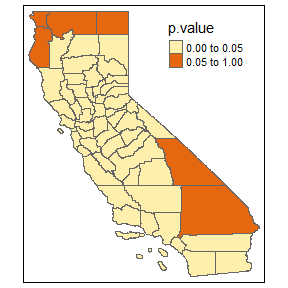If the p-value is lower than the specific level (0.01, 0.05, etc.), significant effects exist. ### 2.3.2 GWPR.plmtest(): Locally Breusch-Pagan Lagrange Multiplier test GWPR.plmtest.resu.F <- GWPR.plmtest(formula = formula.GWPR, data = TransAirPolCalif, index = c("GEOID", "year"), SDF = California, bw = bw.AIC.F, adaptive = F, p = 2, kernel = "bisquare", longlat = F) #> **************** Breusch-Pagan Lagrange Multiplier test in each subsample ******************* #> Formula: pm25 = co2_mean + Developed_Open_Space_perc + Developed_Low_Intensity_perc + Developed_Medium_Intensity_perc + Developed_High_Intensity_perc + Open_Water_perc + Woody_Wetlands_perc + Emergent_Herbaceous_Wetlands_perc + Deciduous_Forest_perc + Evergreen_Forest_perc + Mixed_Forest_perc + Shrub_perc + Grassland_perc + Pasture_perc + Cultivated_Crops_perc + pop_density + summer_tmmx + winter_tmmx + summer_rmax + winter_rmax -- Individuals: 58 #> Bandwidth: 2.010529 ---- Adaptive: FALSE #> Model: Pooling ---- #> If the p-value is lower than the specific level (0.01, 0.05, etc.), significant effects exist. tm_shape(GWPR.plmtest.resu.F$SDF) +
tm_polygons(col = "p.value", breaks = c(0, 0.05, 1))
#> Warning in sp::proj4string(obj): CRS object has comment, which is lost in output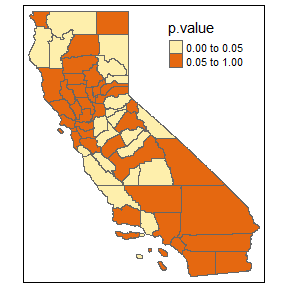If the p-value is lower than the specific level (0.01, 0.05, etc.), significant effects exist.

### 2.3.3 GWPR.phtest(): Locally Hausman test

GWPR.phtest.resu.F <- GWPR.phtest(formula = formula.GWPR, data = TransAirPolCalif, index = c("GEOID", "year"),
SDF = California, bw = bw.AIC.F, adaptive = F, p = 2, effect = "individual",
kernel = "bisquare", longlat = F, random.method = "amemiya")
#> ************************* Hausman Test in each subsample ***************************
#> Formula: pm25  =  co2_mean + Developed_Open_Space_perc + Developed_Low_Intensity_perc + Developed_Medium_Intensity_perc + Developed_High_Intensity_perc + Open_Water_perc + Woody_Wetlands_perc + Emergent_Herbaceous_Wetlands_perc + Deciduous_Forest_perc + Evergreen_Forest_perc + Mixed_Forest_perc + Shrub_perc + Grassland_perc + Pasture_perc + Cultivated_Crops_perc + pop_density + summer_tmmx + winter_tmmx + summer_rmax + winter_rmax -- Individuals: 58
#> Bandwidth: 2.010529 ---- Adaptive: FALSE
#> Model: Fixed Effects vs Random Effects ---- Effect: individual ---- Random Method: amemiya
#> If the p-value is lower than the specific level (0.01, 0.05, etc.), one model is inconsistent.
tm_shape(GWPR.phtest.resu.F$SDF) + tm_polygons(col = "p.value", breaks = c(0, 0.05, 1)) #> Warning in sp::proj4string(obj): CRS object has comment, which is lost in output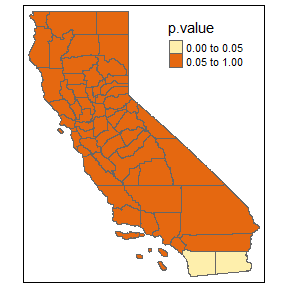If the p-value is lower than the specific level (0.01, 0.05, etc.), one model is inconsistent. Note: Here, we use the “amemiya” random method. Normally, we should use “swar”, but need to change the bandwidth. Because it is just example so we do not do that. ## 2.4 GWPR(): the regression process Now, we come to the most important funciton in this package, GWPR(). Let us look it at first. GWPR(formula = formula, data = data, index = index, SDF = SDF, bw = NULL, adaptive = F, p = 2, effect = "individual", model = c("pooling", "within", "random"), random.method = "swar", kernel = "bisquare", longlat = F) You might know more things about weight least square. Similarly, the GWR equation is as follows ()ref. 3: $y_i = \sum_jX_{ij}\beta_j(p_i)+\epsilon_i$ Here $$p_i$$ is the geographical location of the $$i$$th case. These $$\beta_j(p_i)$$ would themselves contain coefficients to be estimated. If without $$(p_i)$$, the $$\beta$$ should be estimated as follows: $\hat\beta = (X^TX)^{-1}X^Ty$ Now, it should be as follows: $\hat\beta_i = (X^TW_iX)^{-1}X^TW_iy$ This $$W_i$$ is the spatial weights we mention in the beginning of this documents. Now, you know GWR based on OLS. All panel model is just a data transformation, then perform the model use almost the same process. $X' = \begin{cases} X & \text{if model = pooling}\\ X - \bar{X} & \text{if model = FEM}\\ X - \theta\bar{X} & \text{if model = REM}\\ \bar{X} & \text{if model = between}\\ X_t - X_{t-1} & \text{if model = first difference}\\ \end{cases}$ $y' = \begin{cases} y & \text{if model = pooling}\\ y - \bar{y} & \text{if model = FEM}\\ y - \theta\bar{y} & \text{if model = REM}\\ \bar{y} & \text{if model = between}\\ y_t - y_{t-1} & \text{if model = first difference}\\ \end{cases}$ Where $$X'$$ is the data after transformation, $$X$$ is a matrix of original independent variables, $$\bar{X}$$ is the means of all independent variables during all periods. Our GWPR() can solve first three type within the function. “between” and “first difference” can be easily performed, then use our GWPR() with (model = “pooling”) to do. Here, we give an example about GWPR() result.F.AIC <- GWPR(bw = bw.AIC.F, formula = formula.GWPR, data = TransAirPolCalif, index = c("GEOID", "year"), SDF = California, adaptive = F, p = 2, effect = "individual", model = "within", kernel = "bisquare", longlat = F) #> ************************ GWPR Begin ************************* #> Formula: pm25 = co2_mean + Developed_Open_Space_perc + Developed_Low_Intensity_perc + Developed_Medium_Intensity_perc + Developed_High_Intensity_perc + Open_Water_perc + Woody_Wetlands_perc + Emergent_Herbaceous_Wetlands_perc + Deciduous_Forest_perc + Evergreen_Forest_perc + Mixed_Forest_perc + Shrub_perc + Grassland_perc + Pasture_perc + Cultivated_Crops_perc + pop_density + summer_tmmx + winter_tmmx + summer_rmax + winter_rmax -- Individuals: 58 #> Bandwidth: 2.010529 ---- Adaptive: FALSE #> Model: within ---- Effect: individual #> The R2 is: 0.896960596779094 #> Note: in order to avoid mistakes, we forced a rename of the individuals'ID as "id". summary(result.F.AIC$SDF$Local_R2) #> Min. 1st Qu. Median Mean 3rd Qu. Max. #> 0.2899 0.4272 0.4676 0.4928 0.5375 0.8467 tm_shape(result.F.AIC$SDF) +
tm_polygons(col = "Local_R2", pal = "Reds",auto.palette.mapping = F,
style = 'cont')
#> Warning: The argument auto.palette.mapping is deprecated. Please use midpoint
#> for numeric data and stretch.palette for categorical data to control the palette
#> mapping.
#> Warning in sp::proj4string(obj): CRS object has comment, which is lost in output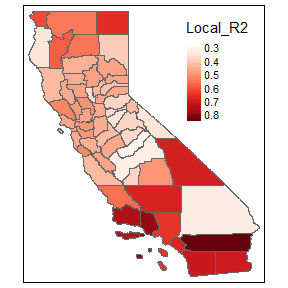Look, the $$R^2$$ is 0.8969606, while the panel liner regression is 0.48772. BAM!!!
Note:Our model include SE and t-value of local coefficients, you can check the significance.

# END

Thank for your cooperation, we are going to write an article about this package. After it is publsihed, we will update the reference of all. If you could cite our article we would really appreciate.

## Archive in GitHub:

https://github.com/MichaelChaoLi-cpu/GWPR.light

## BugReports:

If you find bugs or have other ideas, be free to contact us. https://github.com/MichaelChaoLi-cpu/GWPR.light/issues

# Reference

1. Beenstock, M., Felsenstein, D., 2019. The econometric analysis of non-stationary spatial panel data. Springer.
2. Fotheringham, A., Brunsdon, C., Charlton, M., 2002. Geographically Weighted Regression: The Analysis of Spatially Varying Relationships. John Wiley & Sons 13.
3. Brunsdon, C., Fotheringham, S., Charlton, M., 1998. Geographically Weighted Regression. Journal of the Royal Statistical Society: Series D (The Statistician) 47, 431-443.